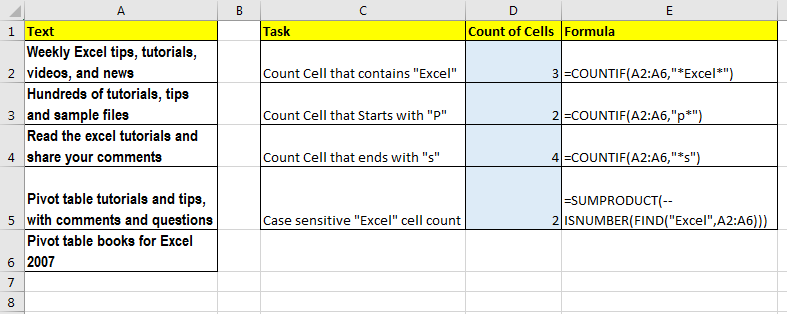# How to Count Cells That Contain Specific Text in Excel

So, the objective is count every cell that contains a specific text. Right? Thats no big deal. We can just use COUNTIF function to count cells that contains specific text with help of wild card character asterisk (*).Generic Formula to Count Cells That Contains Specific Text.

=COUNTIF(range,”*text*”)

#### Examples

To count all cell that contain “ExcelTip” in range A1:A10, we will write this formula:

=COUNTIF(A1:A10,”*ExcelTip*”)

=COUNTIF(A1:A10,”a*”)

To Count Cell that ends with “etc” write this COUNTIF formula

=COUNTIF(A1:A10,”*etc”)

Since COUNTIF is not case sensitive. It counts all cells that contain given text, irrespective of their case. Then how do we count case sensitive text?

#### HOW TO COUNT CELL CONTAINING CASE SENSITIVE SPECIFIC TEXT

To count cells with case sensitive condition we would use SUMPRODUCT, FIND and ISNUMBER function.

Generic Formula

=SUMPRODUCT(--(ISNUMBER(FIND(text,range))))

For example, if you want to count cells that contain exactly “Excel” not “excel write this formula:

=SUMPRODUCT(--(ISNUMBER(FIND(“Excel”,range))))

The FIND function will return array of found locations of “Excel” and #VALUE errors.
ISNUMBER will then check each element in array, if its a number or not and will return an array of TRUE and FALSE. Means if text is found then TRUE else FALSE.
-- unary operator will convert TRUE into 1 and FALSE into 0.
Finally, SUMPRODUCT will sum this array and we have number of cell that contains “Excel

Popular Articles:

50 Excel Shortcuts to Increase Your Productivity

How to use the VLOOKUP Function in Excel

How to use the COUNTIF function in Excel

How to use the SUMIF Function in Excel

Terms and Conditions of use

The applications/code on this site are distributed as is and without warranties or liability. In no event shall the owner of the copyrights, or the authors of the applications/code be liable for any loss of profit, any problems or any damage resulting from the use or evaluation of the applications/code.